# CVCX Online Main Page

CVCX Online consists of the sim Viewers and 12 calculators as follows:

## CVCX Online Data Viewer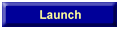150,000 2,000,000,000 round sims with different combinations of decks, rules and strategies reside on the CVCX Online server. The viewer can be used to look at the sim results. For each sim, you can change the spread, risk and other variables and results along with the optimal betting ramp for your settings will be instantly displayed. Full CVCX functionality (reports, charts, simulation, custom bets, etc) cannot be made available due to Web limitations. But, I believe the data most commonly used is available. Click Launch to invoke the viewer. For more info on CVCX, see CVCX Pages.

## CVCX Online Chart Viewer21,000 graphs with different combinations of decks, rules and strategies also reside on the CVCX Online server. You can change the strategy, decks, rules and spread and charts can be displayed of SCORE, Advantage and N0 for all reasable penetrations assuming optimal betting at a risk of ruin of 13.5%. About 4,000,000 data points have been plotted. This is a tiny fraction of all possible siuations; but should provide a good view of the effect of various variables on performance. Click Launch to invoke the viewer. Sorry, this function is only available to CVCX users.

## Calculators

Twelve Blackjack calculators can be found below to answer various 'what if' questions. Win Rates and Standard Deviations used in these calculators can be found in the CVCX Online Viewer. Most of the methodologies used are either in or based on formulae in Don Schlesinger’s Blackjack Attack and page references are supplied below. Pick the desired calculator and hit the Launch button.

### RiskRisk given no goal and no time constraint - This is the Simple Risk of Ruin formula on Blackjack Attack page 112. The result is the risk of ruin with no limit on the number of hands and no quit point. Simply set the bankroll.Risk given no goal but a time constraint - This is the trip ruin formula in Blackjack Attack page 132. Risk is still calculated, but for a specified number of hands. The risk is lower than with the above calculator since you have a quit point.Risk given a goal and time constraint - This is called a double barrier formula and is found on Blackjack Attack page 137. We are calculating the risk of bankruptcy before hitting a goal or playing a number of hands. Here, you set a point at which you will stop playing. The risk is lower than the above calculator since you will stop playing if you hit one of two quit points: Time or Goal.Risk given a goal and no time constraint - This is a modification of the above with the number of hands removed. It can be found on Blackjack Attack page 139. Here you quit if you hit a goal, but there is no limit on number of hands.

### GoalProbability of reaching a goal before going bankrupt given a time constraint - This formula is found on Blackjack Attack page 136. Here you specify the maximum number of hands you will play, a bankroll and a goal. The result is the probability of reaching that goal before going bankrupt or hitting the specified number of hands.Probability of reaching a goal before going bankrupt with no time constraint - This formula is found on Blackjack Attack page 138. This is the same as the above calculator except that there can be an unlimited number of hands. Therefore, the probability of reaching the goal is a little higher.

### ResultsRange of Probable Results - Here, you specify the number of hands you will play and a probability and the range of your results is calculated. For example, if you specify 95%, and the answer is -664 to 1346, then 95% of the time your winnings will be somewhere between -664 and 1,346 units. The higher the percent that you specify, the wider the range.What results can I expect given time and probability of reaching goal without ruin - This is the formula on page 137 solved for goal. Your probable results are calculated. You must give a probability. For example, if you specify 98%, you will hit the calculated goal 98% of the time without going bankrupt within the specified number of hands.

### ActualProbability of your actual results - Here you can enter the actual casino results from a trip to see how far you were from the expected results. Set the number of hands played and how much you won or lost. If the answer is 50%, you won the amount that you should expect. If you won more than you expected, the answer will be the percentage of times that you should expect at least that result.

### BankrollWhat is the required bankroll given a goal and time constraint - How much do you need to avoid bankruptcy? This is the formula on page 137 solved for bankroll. Here, you enter the number of hands you will play on a trip, your goal and the desired probability of reaching that goal. The required bankroll is calculated.What is the required bankroll given a goal and no time constraint - How much do you need to avoid bankruptcy? This is the formula on page 139 solved for bankroll. This is the same as the above calculator, except there is no limit on the number of hands played.

### TimeHands required given goal and desired probability - This is the formula on page 137 solved for time. Here, you calculate the needed number of hands.

Share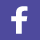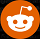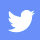News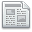Forum/Blog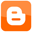Fav/EMailGambling problem, contact the National Council on Problem Gambling.